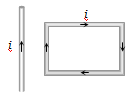A beam of ions with velocity  enters normally into a uniform magnetic field of $4×{10}^{-2}$tesla. If the specific charge of the ion is $5×{10}^{7}$ C/kg , then the radius of the circular path described will be :

(a) 0.10 m              (b)  0.16 m

(c)  0.20 m              (d) 0.25 m

Concept Questions :-

Lorentz force
High Yielding Test Series + Question Bank - NEET 2020

Difficulty Level:

If the direction of the initial velocity of the charged particle is perpendicular to the magnetic field, then the orbit will be
or
The path executed by a charged particle whose motion is perpendicular to magnetic field is :

(a) A straight line                                (b) An ellipse
(c) A circle                                          (d) A helix

Concept Questions :-

Lorentz force
High Yielding Test Series + Question Bank - NEET 2020

Difficulty Level:

A proton and an $\mathrm{\alpha }-$ particle enter a uniform magnetic field perpendicularly with the same speed. If proton takes 25 $\mathrm{\mu }$ sec to make 5 revolutions, then the periodic time for the $\mathrm{\alpha }-$particle would be :

(a) 50 $\mathrm{\mu }$ sec                (b) 25 $\mathrm{\mu }$ sec

(c) 10 $\mathrm{\mu }$ sec                 (d) 5 $\mathrm{\mu }$ sec

Concept Questions :-

Lorentz force
High Yielding Test Series + Question Bank - NEET 2020

Difficulty Level:

An $\mathrm{\alpha }-$ particle travels in a circular path of radius 0.45 m in a magnetic field  with a speed of  . The period of revolution of the $\mathrm{\alpha }-$ particle is :

(a)   sec          (b)  $1.1×{10}^{-6}$ sec

(c)  $1.1×{10}^{-7}$ sec           (d)  $1.1×{10}^{-8}$ sec

Concept Questions :-

Lorentz force
High Yielding Test Series + Question Bank - NEET 2020

Difficulty Level:

A deutron of kinetic energy 50 keV is describing a circular orbit of radius 0.5 metre in a plane perpendicular to magnetic field $\stackrel{\to }{\mathrm{B}}$. The kinetic energy of the proton that describes a circular orbit of radius 0.5 metre in the same plane with the same $\stackrel{\to }{\mathrm{B}}$ is :

(a) 25 kev                           (b) 50 kev

(c) 200 kev                          (d) 100 kev

Concept Questions :-

Lorentz force
High Yielding Test Series + Question Bank - NEET 2020

Difficulty Level:

A rectangular loop carrying a current i is situated near a long straight wire such that the wire is parallel to the one of the sides of the loop and is in the plane of the loop. If a steady current I is established in wire as shown in figure, the loop will(a) Rotate about an axis parallel to the wire

(b) Move away from the wire or towards right

(c) Move towards the wire

(d) Remain stationary

Concept Questions :-

Current carrying loop : Force and torque
High Yielding Test Series + Question Bank - NEET 2020

Difficulty Level:

Two thin long parallel wires separated by a distance b are carrying a current i amp each. The magnitude of the force per unit length exerted by one wire on the other is

(a) $\frac{{\mu }_{0}{i}^{2}}{{b}^{2}}$                                  (b) $\frac{{\mu }_{0}{i}^{2}}{2\mathrm{\pi b}}$

(c) $\frac{{\mu }_{0}i}{2\mathrm{\pi b}}$                                   (d) $\frac{{\mu }_{0}i}{2{\mathrm{\pi b}}^{2}}$

Concept Questions :-

Force between current carrying wires
High Yielding Test Series + Question Bank - NEET 2020

Difficulty Level:

To make the field radial in a moving coil galvanometer :

(a) The number of turns in the coil is increased

(b) Magnet is taken in the form of horse-shoe

(c) Poles are cylindrically cut

(d) The coil is wounded on the aluminum frame

Concept Questions :-

Moving coil galvanometer
High Yielding Test Series + Question Bank - NEET 2020

Difficulty Level:

In a moving coil galvanometer, the deflection of the coil $\theta$ is related to the electrical current i by the relation

(a) $i\propto \mathrm{tan}\theta$                  (b) $i\propto \theta$

(c) $i\propto {\theta }^{2}$                     (d) $i\propto \sqrt{\theta }$

Concept Questions :-

Moving coil galvanometer
High Yielding Test Series + Question Bank - NEET 2020

Difficulty Level:

A moving coil galvanometer has N number of turns in a coil of effective area A, it carries a current I. The magnetic field B is radial. The torque acting on the coil is

(a) $N{A}^{2}{B}^{2}I$                           (b) $NAB{I}^{2}$

(c) ${N}^{2}ABI$                             (d) $NABI$

Concept Questions :-

Moving coil galvanometer